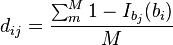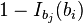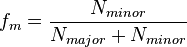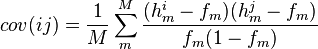ANGSD: Analysis of next generation Sequencing Data

Latest tar.gz version is (0.938/0.939 on github), see Change_log for changes, and download it here.

# single read sampling approach for PCA or MDS

This function is new and works from version 0.912 and in the latest developmental version from github

For the PCA / MDS methods you should called SNP sites (use PCA if you do not want to call SNPs). SNPs can be called based on genotype likelihoods (see SNP_calling) or you can give the variable sites you want analysis using the -sites options.

# Brief Overview

./angsd -doIBS
-> angsd version: 0.911-26-gf1cb0e0-dirty (htslib: 1.3-1-gc72ae90) build(Apr 27 2016 11:15:33)
-> Analysis helpbox/synopsis information:
-> Command:
../angsd/angsd -doIBS 	-> Wed Apr 27 12:38:35 2016
--------------
abcIBS.cpp:
-doIBS	0
(Sampling strategies)
0:	 no IBS
1:	 (Sample single base)
2:	 (Concensus base)
-doCounts	0	Must choose -doCount 1
Optional
-minMinor	0	Minimum observed minor alleles
-minFreq	0.000	Minimum minor allele frequency
-output01	0	output 0 and 1s instead of based
-maxMis		-1	Maximum missing bases (per site)
-doMajorMinor	0	use input files or data to select major and minor alleles
-makeMatrix	0	print out the ibs matrix
-doCov		0	print out the cov matrix


## Options

-doIBS [int]

Print a single base from each individual at each position. 1: random sampled read. 2: Consensus base. If you do not want to print out every site then use -1 or -2.

-doCounts [int]

Method requeres counting the different bases at each position. Therefore, -doCounts 1 must be used

-doMajorMinor [int]

The covariance matrix can only be calculated for diallelic sites. Therefore, choose a methods for selecting the major and minor allele (see Inferring_Major_and_Minor_alleles). This can also be use if you only want to make this assumption for the IBS matrix or only want to print out bases that are either the major or minor.

-minMinor [int]

Minimum observed minor alleles. The default in 0. If you do not use -doMajorMinor then the number of minor alleles are the sum of the 3 most uncommon alleles.

-minFreq [float]

Minimum minor allele frequency based on the sampled bases. The default in 0. If you do not use -doMajorMinor then the frequency is the sum of the frequencies of the 3 most uncommon alleles.

-output01 [int]

output the samples reads as 0 (for major) and 1s (for non major) instead of actual base

-maxMis [int]

Maximum missing bases (per site) i.e. is the maximum number of allowed non-major/minor sampled bases

-makeMatrix [int]

1 prints out the pairwise IBS matrix. This is the avg. distance between pairs of individuals. Distance is zero if the base in the same and 1 otherwise. You can use this for MDS (see below)

-doCov [int]

1 print out the covariance matrix which can be used for PCA (see below). You should use the -minFreq option to avoid sites with low allele frequency.

### run example

./angsd -bam all.files -minMapQ 30 -minQ 20 -GL 2  -doMajorMinor 1 -doMaf 1 -SNP_pval 2e-6 -doIBS 1 -doCounts 1 -doCov 1 -makeMatrix 1 -minMaf 0.05 -P 5


This will produce the output (see below) which includes pairwise differences (.ibsMat) and the covariance matrix (.covMat). These can be used for MDS and PCA respectively (see R example below). Note that only the PCA method require SNP calling and allele frequency estimation.

## Output

### sampled bases *ibs.gz

This function will print the sampled based *ibs.gz.

Example of output *.ibs.gz with -doMajorMinor>0 and -output01 1

chr     pos     major   minor   ind0    ind1    ind2    ind3    ind4    ind5    ind6    ind7
1       14000873        A       G       0       1       1       1       1       1       1
1       14001018        C       T       0       1       1       1       1       1       1
1       14001867        G       A       0       1       1       1       1       0       1
1       14002342        T       C       1       1       1       1       1       -1      1
1       14002422        T       A       0       1       1       1       1       0       -1
1       14003581        T       C       0       1       1       1       1       1       1
1       14004623        C       T       0       1       1       1       1       0       1
1       14006543        T       G       0       -1      1       1       1       0       1
1       14007493        G       A       0       0       1       -1      1       0       1
1       14007558        T       C       0       0       1       1       -1      -1      1
1       14007649        A       G       0       1       1       1       1       0       1
1       14008269        A       G       1       1       0       -1      1       -1      1


Example of output *.ibs.gz with -doMajorMinor>0 and -output01 0

chr     pos     major   minor   ind0    ind1    ind2    ind3    ind4    ind5    ind6    ind7
1       13116   G       T       N       G       T       T       N       G       N       T
1       13118   G       A       N       G       A       A       N       G       N       A
1       14930   A       G       G       G       G       A       N       N       A       N
1       15211   T       G       N       G       T       G       N       N       N       G
1       54490   A       G       N       G       N       G       N       N       N       N
1       54716   T       C       T       C       C       C       T       N       N       N
1       58814   A       G       N       G       N       G       G       G       N       N
1       62777   T       A       N       N       A       N       A       A       A       N
1       63268   C       T       N       T       N       T       C       N       T       N
1       63671   A       G       N       G       N       N       G       G       G       N
1       69428   G       T       N       G       T       N       N       T       T       N
1       69761   T       A       A       A       T       A       N       A       N       N


Example of output *.ibs.gz with -doMajorMinor 0 and -output01 0

chr     pos     major   ind0    ind1    ind2    ind3    ind4    ind5    ind6    ind7    ind8
1       13116   T       N       G       T       T       N       G       N       T       T
1       13118   A       N       G       A       A       N       G       N       A       A
1       14930   A       G       G       G       A       N       N       A       N       G
1       15211   G       N       G       T       G       N       N       N       G       G
1       54490   G       N       G       N       G       N       N       N       N       A
1       54716   C       T       C       C       C       T       N       N       N       C
1       58814   G       N       G       N       G       G       G       N       N       G
1       62777   A       N       N       A       N       A       A       A       N       A
1       63268   T       N       T       N       T       C       N       T       N       N
1       63336   C       C       C       C       C       C       N       C       N       N
1       63671   G       N       G       N       N       G       G       G       N       N


chr is the chromosome

pos is the position

major is the major allele

minor is the minor allele. Needs -doMajorMinor

indX is the sampled base for individual number X. if -output01 1 then it is 1 for major, 0 for non major and -1 for missing

### sample based IBS matrix *.ibsMat

This function will print the pairwise IBS distance

Example of output *.ibsMat with -makeMatrix 1

0.000000        0.510638        0.606383        0.595745        0.545455        0.428571
0.510638        0.000000        0.154639        0.154639        0.108911        0.408602
0.606383        0.154639        0.000000        0.121212        0.137255        0.489362
0.595745        0.154639        0.121212        0.000000        0.106796        0.484211
0.545455        0.108911        0.137255        0.106796        0.000000        0.404040
0.428571        0.408602        0.489362        0.484211        0.404040        0.000000
0.577320        0.121212        0.181818        0.171717        0.097087        0.473684
0.536082        0.090000        0.138614        0.118812        0.047619        0.428571
0.262500        0.571429        0.702381        0.694118        0.632184        0.353659
0.458333        0.383838        0.484848        0.494949        0.398058        0.368421


Nind x Nind matrix with pairwise IBS distance

### sample based covariance matrix *.covMat

This function will print the covariance matrix based on a single sampled read

Example of output *.covMat with -doCov 1


1.098251        -0.026225       -0.005617       -0.014726       -0.022438       -0.021786
-0.026225       1.115986        -0.017167       0.000735        -0.017163       -0.016899
-0.005617       -0.017167       1.074779        -0.015685       -0.019819       -0.015473
-0.014726       0.000735        -0.015685       1.072853        -0.013641       -0.007789
-0.022438       -0.017163       -0.019819       -0.013641       1.094612        -0.016045
-0.021786       -0.016899       -0.015473       -0.007789       -0.016045       1.059264
-0.005831       -0.009854       -0.001269       -0.002362       -0.018479       -0.011942
-0.015399       -0.020010       -0.001296       -0.022947       -0.006515       -0.003938
-0.001730       -0.040534       -0.002295       -0.017442       -0.024194       -0.007469
-0.016094       -0.015303       -0.018302       -0.022502       -0.030503       -0.001208
-0.122045       -0.106068       -0.103089       -0.104443       -0.110237       -0.103610
-0.106553       -0.100202       -0.104754       -0.109399       -0.107645       -0.111665
-0.108945       -0.102440       -0.105292       -0.101372       -0.107110       -0.106639


Nind x Nind covariance matrix

## Model

### IBS

pairwise distance between individuals$d_{ij} = \frac{\sum_m^M 1-I_{b_j}(b_i)}{M}$ where M in the number of sites with a read for both individuals.$1-I_{b_j}(b_i)$ is the indicator function which is equal to one with the two individuals i and j have the same base and zero otherwise

### Covariance

Allele frequency based on single reads.$f_{m} = \frac{N_{minor}}{N_{major} + N_{minor}}$$cov(ij) = \frac{1}{M}\sum_m^M \frac{ (h^i_m-f_m)(h^j_m-f_m) }{f_m(1-f_m)}$

where M in the number of sites with a read for both individuals.$h^i_m$ is 1 if individuals i for site m has the major allele and zero otherwise

# MDS/PCA using R

## MDS
name <- "angsdput.ibsMat"
mds <- cmdscale(as.dist(m))
plot(mds,lwd=2,ylab="Dist",xlab="Dist",main="multidimensional scaling",col=rep(1:3,each=10))

name <- "angsdput.covMat"
e <- eigen(m)
plot(e\$vectors[,1:2],lwd=2,ylab="PC 2",xlab="PC 2",main="Principal components",col=rep(1:3,each=10),pch=16)



# other fun stuff

## heatmap / clustering / trees
name <- "angsdput.ibsMat" # or covMat